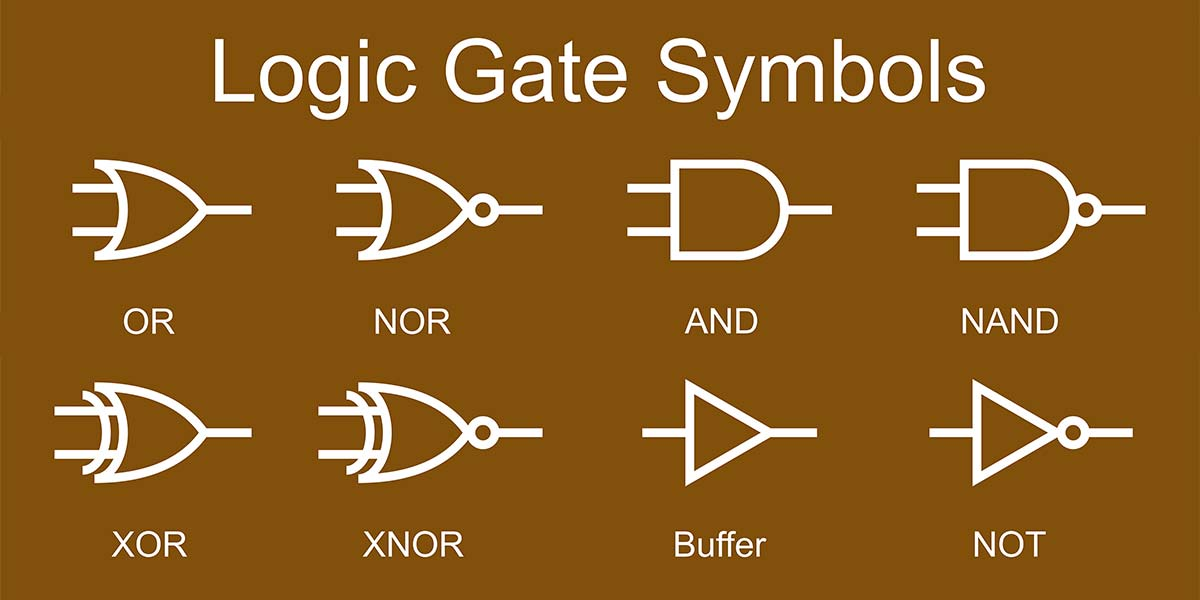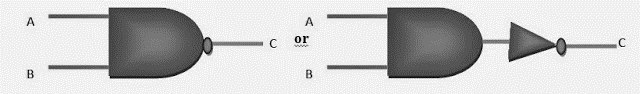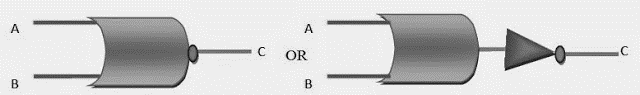# Alternative Logic Gate

Welcome to Class !!

In today’s Computer Science class, We will be learning about Alternative Logic Gates. We hope you enjoy the class!These are gates that are formed from a combination of two logic gates. There are two types of alternative logic gates:

#### NAND GATE

A NAND gate is the combination of an AND gate and NOT gate. It operates the same as an AND gate but the output will be the opposite. Remember, the NOT gate does not always have to be the output leg; it could be used to invert an input signal also.LOGIC SYMBOL FOR THE “NAND” GATE

Notice the circle on output C.

###### OUTPUT
A B C
0 0 1
0 1 1
1 0 1
1 1 0

NAND GATE EQUATION

The NAND gate operation can also be expressed by a Boolean algebra equation. For a 2 – input NAND gate, the equation is:

This equation read X equal to A and B NOT, which simply means that the output of the gate is not a logic 1 when A and B inputs are their 1 states.

#### NOR GATE

A NOR gate is the combination of both an OR gate and NOT gate. It operates the same as an OR gate, but the output will be the opposite.A B C
0 0 1
0 1 0
1 0 0
1 1 0

###### NOR GATE EQUATION

The NOR gate operation can also be expressed by a Boolean algebra equation. For a 2 – input NAND gate, the equation is:

The expression is the same as the OR gate with an overbar above the entire portion of the equation representing the input. This equation read X equal to A or B NOT, which simply means that the output of the gate is not a logic 1 when A or B are in their 1 states.

##### USES OF LOGIC GATES

Logic gates are in fact the building block of digital electronics, they are formed by the combination of transistors (either BJT or MOSFET) to realise some digital operations like logical OR, NOT, AND etc. Every digital product like computers, mobile phones, calculators, even digital watches contains logical gates.

##### XOR GATE

The XOR (exclusive – OR) gate acts in the same way as the logical “either-or”. The output is “True” if either but not both, of the inputs, are “true”. The output is “false” or if both inputs are “true”.LOGIC SYMBOL FOR “XOR” GATE

TRUTH TABLE FOR THE “XOR” GATE

 INPUT INPUT OUTPUT A B Y 0 0 0 0 1 1 1 0 1 1 1 0

###### XOR COMPARATOR

Comparator is a combinational logic circuit that compares the magnitudes of two binary quantities to determine which one has the greater magnitude. In order word, comparator determines the relationship of two binary quantities. An XOR can be used as a basic comparator.

As you can see, the only difference between these two symbols is that the XNOR has a circle on its output to indicate that the output is inverted.One of the most common uses for XOR gates is to add two binary numbers. For this operation to work, the XOR gate must be used in combination with an AND gate.

To understand how the circuit works, review how binary addition works:

0 + 0 = 0

0 + 1 = 1

1 + 0 = 1

1 + 1 = 10

If you wanted, you could write the results of each of the preceding addition statements by using two binary digits, like this:

0 + 0 = 00

0 + 1 = 01

1 + 0 = 01

1 + 1 = 10

When results are written with two binary digits, as in this example, you can easily see how to use an XOR and an AND circuit in combination to perform binary addition.

If you consider just the first binary digit of each result, you’ll notice that it looks just like the truth table for an AND circuit and that the second digit of each result looks just like the truth table for an XOR gate.

The adder circuit has two outputs. The first is called the Sum, and the second is called the Carry. The Carry output is important when several adders are used together to add binary numbers that are longer than 1 bit.###### EVALUATION
1. Describe Alternative Logic Gates with examples and how they are achieved

We have come to the end of this class. We do hope you enjoyed the class?

Should you have any further question, feel free to ask in the comment section below and trust us to respond as soon as possible.

In our next class, we will continue learning about Alternative Logic Gates. We are very much eager to meet you there.

Are you a Parent? Share your quick opinion and win free 2-month Premium Subscription

Don`t copy text!